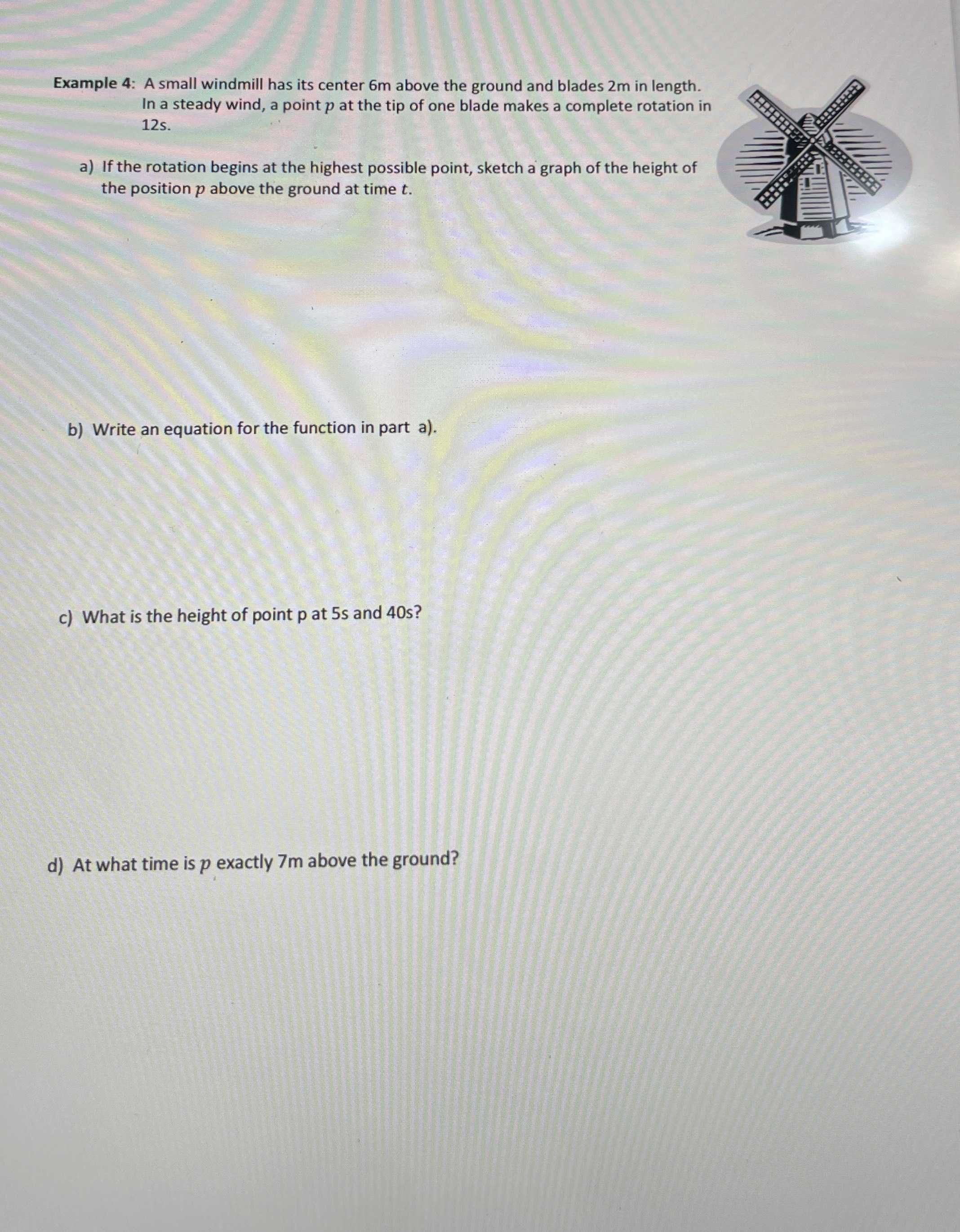### Still have math questions?

Algebra
QuestionExample 4: A small windmill has its center $$6 m$$ above the ground and blades $$2 m$$ in length. In a steady wind, a point $$p$$ at the tip of one blade makes a complete rotation in $$12 s$$ .

a) If the rotation begins at the highest possible point, sketch a graph of the height of the position $$p$$ above the ground at time $$t$$ .

b) Write an equation for the function in part a).

c) What is the height of point $$p$$ at $$5 s$$ and $$40$$ s?

d) At what time is $$p$$ exactly $$7 m$$ above the ground?

b. $$T = 125 , w = \frac { 1 } { 6 } \pi$$
whent $$= 0 , h = 8 m$$
when $$t = 65 h = 4 m$$
$$h = 2 \cos ( \frac { 1 } { 6 } \pi t ) + 6$$